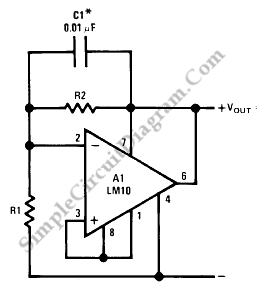# LM10 Shunt Regulator

Shunt regulator is equivalent with zener diode regulator, where you can connect the circuit in series with a resistor and tap the output at the junction. With LM10, we can build more stable regulator (compared to zener diode version). Here is the schematic diagram of the circuit:The nominal voltage of the circuit is the internal voltage reference multiplied by [R1/(R1+R2)]. The internal voltage reference for LM10 is 200 mV. [Circuit’s schematic diagram source: National Semiconductor Application Note]